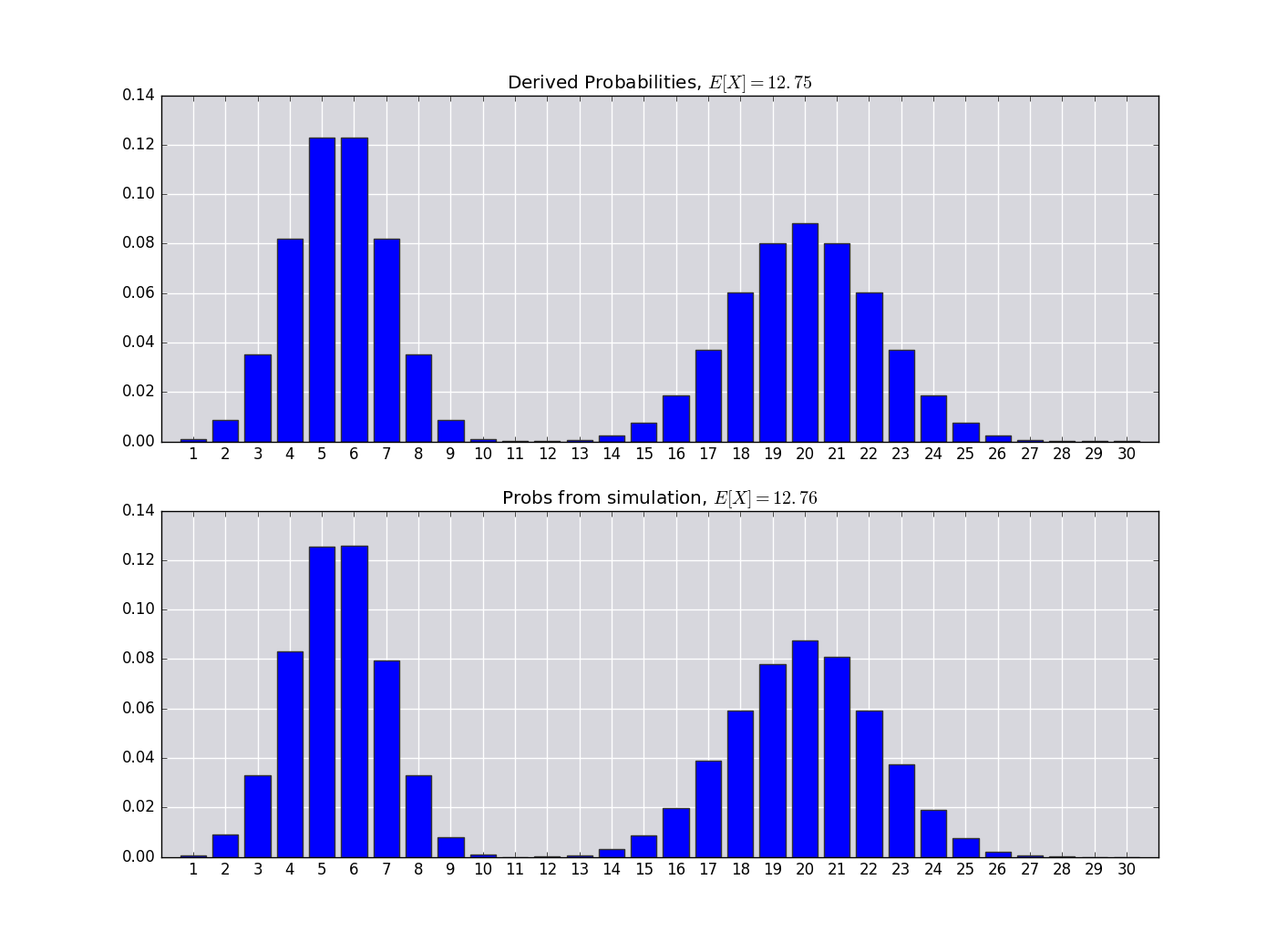You are one of 30 team owners in a professional sports league. In the past, your league set the order for its annual draft using the teams’ records from the previous season — the team with the worst record got the first draft pick, the team with the second-worst record got the next pick, and so on. However, due to concerns about teams intentionally losing games to improve their picks, the league adopts a modified system. This year, each team tosses a coin. All the teams that call their coin toss correctly go into Group A, and the teams that lost the toss go into Group B. All the Group A teams pick before all the Group B teams; within each group, picks are ordered in the traditional way, from worst record to best. If your team would have picked 10th in the old system, what is your expected draft position under the new system?

Denote the following events:

\begin{align*} \{X=j\} &\Leftrightarrow \{\text{Your team gets draft position, }j\} \\ \{N=n\} &\Leftrightarrow \{n\text{ teams called their coin toss correctly.}\} \\ \{W / L\} &\Leftrightarrow \{\text{Your team wins / loses its coin toss.}\} \end{align*}

Thus, we want to know $E[X]$. First,

\begin{align*} P(X=j) = \sum_{n=0}^{30} &\left[P(X=j|N=n,W)P(N=n, W)\right. \\ +&\left.P(X=j|N=n,L)P(N=n,L)\right] \tag{1} \end{align*}

$N$ is clearly binomial, and $P(W|N=n) = \frac{n}{30}$, so that

$P(W, N=n) = P(W|N=n)P(N=n) = \frac{n}{30} {30 \choose n} \left(\frac{1}{2}\right)^{30}$

$P(L,N=n)$ also follows from the above. Finally the last pieces are $P(X=j|N=n,W)$ and $P(X=j|N=n,L)$. These distributions are obtained by counting. In the first, the probability is zero if $j \gt n$ or if $n \gt 10$ and $j \gt 10$. Otherwise, to be in draft position $j$, we must choose $j-1$ of the 9 teams with draft positions greater than ours, and we must choose $n-j$ of the 20 teams with draft positions lower than our own. The total number of choices is found by choosing $n-1$ from among the 29 remaining teams (since it is given that we won our coin toss). Thus,

$P(X=j | N=n, W) = \begin{cases} 0 & j \gt n \\ 0 & n \gt 10\text{ and }j \gt 10 \\ \frac{\binom{9}{j-1} \binom{20}{n-j}}{\binom{29}{n-1}} & \text{otherwise} \end{cases}$

Note that this distribution is not defined for $n=0$. By similar arguments, we can obtain:

$P(X=j | N=n, L) = \begin{cases} 0 & n \leq j \\ 0 & n \lt 20\text{ and }j \gt n+10 \\ \frac{\binom{9}{j-1-n} \binom{20}{30-j}}{\binom{29}{20-n}} & \text{otherwise} \end{cases}$

Now we have all the pieces to plug into $(1)$, and we could probably whittle it down to a simplified form. But I don’t feel like whittling. Instead, I’ll write a script to compute the expectation and a simulation function to compare.

Now let’s compare our derived results with the empirical ones from the simulation function:

The simulated results agree with the above derived ones:Notice that if your team wins its coin toss, you’ll likely get a much better position over your original 10th place draft position, and if you lose the coin toss, you’ll likely get a much worse position. On average, though, you’ll only do a little be worse (12.75).